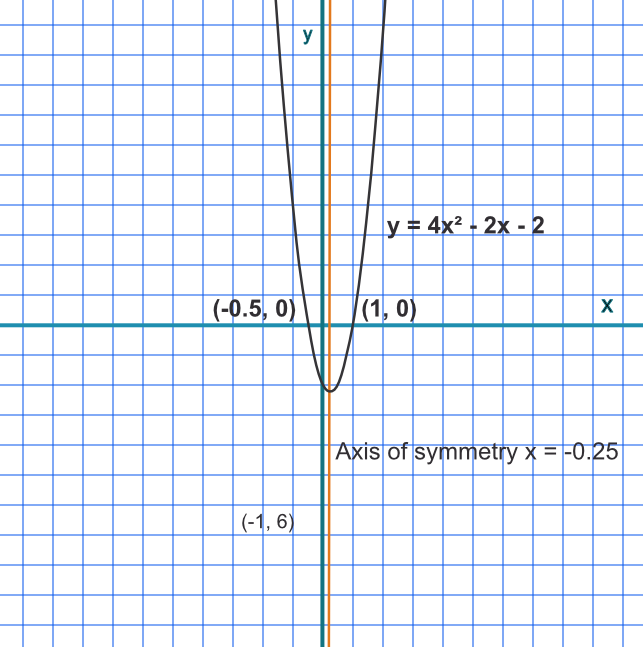Axis of Symmetry for a Quadratic

## Axis of Symmetry for a Quadratic

The Axis of Symmetry lies halfway between the two roots for a quadratic graph.

## Example 1

The function 4x^2-2x-2 factorises to (2x+1)(2x-2).

Give the axis of symmetry to 2 decimal places.

The roots are at x=-frac(1)(2) and x=1

The Axis of Symmetry is halfway between at x=0.25Answer: x=0.25

## Example 2

If the Axis of Symmetry for a quadratic is x=-3.5, and one root is x=-5, what is the other root given that the root must be greater than -3?

The distance between the root and the axis is 1.5. As the question states that the root is greater than -3, then -3.5 + 1.5 = 2.

Answer: x=-2